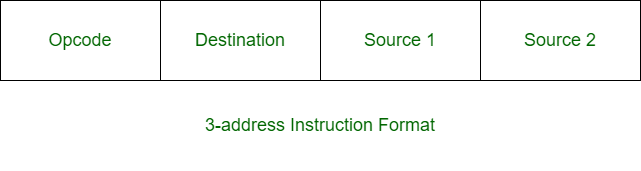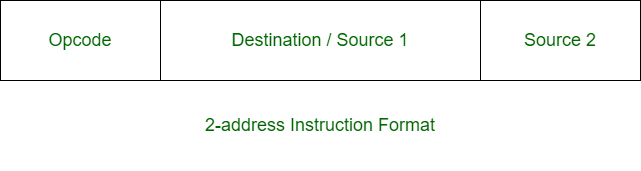• Last Updated : 20 Jun, 2020

Prerequisite – Instruction Formats
Three-address instruction is a format of machine instruction. It has one opcode and three address fields. One address field is used for destination and two address fields for source.Attention reader! Don’t stop learning now.  Practice GATE exam well before the actual exam with the subject-wise and overall quizzes available in GATE Test Series Course.

Learn all GATE CS concepts with Free Live Classes on our youtube channel.

Example –

`X = (A + B) x (C + D) `

Solution:

```ADD R1, A, B      R1 <- M[A] + M[B]
ADD R2, C, D      R2 <- M[C] + M[D]
MUL X, R1, R2     M[X] <- R1 x R2 ```

Two-address instruction is a format of machine instruction. It has one opcode and two address fields. One address field is common and can be used for either destination or source and other address field for source.Example –

`X = (A + B) x (C + D) `

Solution:

```MOV R1, A      R1 <- M[A]
ADD R1, B      R1 <- R1 + M[B]
MOV R2, C      R2 <- M[C]
ADD R2, D      R2 <- R2 + D
MUL R1, R2     R1 <- R1 x R2
MOV X, R1      M[X] <- R1 ```